# Analytical and Numerical Analysis of Thermal Transfer in Disc Brake

Analytical and Numerical Analysis of Thermal Transfer in Disc Brake
Laboratory LMEET, FST of the University Hassan 1st, Settat 26000, Morocco

Laboratory LME, University Chouaib Doukkali, El Jadida 24000, Morocco

Laboratory CAMEL, UQAT J9X 5E4, Canada

Corresponding Author Email:
Mohamedmaniana@hotmail.com
Page:
693-698
|
DOI:
https://doi.org/10.18280/ijht.400305
26 March 2022
|
Accepted:
20 June 2022
|
Published:
30 June 2022
| Citation

OPEN ACCESS

Abstract:

Research in the field of transient thermal transfer has a great importance, both at the basic level and at the digital level. The automotive, railway and air industries focus on braking’s problem, to improve the quality and efficiency of this one. The problem of braking is related to an intensive heat transfer, which depends on several factors, as the geometrical design of the disc, the material it’s made and the nature of cooling. This work involves the analysis and characterization of this transfer, by conduction between the disc and the brake pads, for the automotive system. This work made it possible to make a comparative study, of the evolution of the temperature in the brake disc during the braking operation, between the analytical solution and the numerical solution of the resolution of the equation of the heat in the disc and pad of a braking system under real conditions. This study shows a satisfactory agreement between the two methods for the evaluation of the maximum temperature reached in the disc/pad contact surface during the braking operation. In addition, the knowledge of the temperatures reached in the elements in contact, is important to understand the phenomena taking place within the interface.

Keywords:

brake system, disc, pads, evolution temperature, friction contact, heat flow, simulation, numerical analysis, analytical solution

1. Introduction

The braking mechanism consists of the conversion of the kinetic energy developed by the vehicle into thermal energy dissipated by friction at the brake disc and pads contact.

In order to secure vehicles and ensure the comfort of passengers, brake disc construction technology has been the subject of numerous studies in the automotive, trains and aircraft industry.

In the thermal aspect of the braking domain, several theoretical and analytical studies have been carried out which have established models of temperature calculation in the friction contact surface, well known in the scientific field, most of which are based on the principle of the thermal contact resistance. These models are classified according to the nature of contact: perfect or smooth contact, imperfect contact or with asperities. Among which Majcherczak et al. , in his bibliographic study in 2003, cited the following three models.

2. Numerical Models

2.1 Model of Newcomb

In his study in 1959, Newcomb  considers a unidirectional heat flow through four infinite surfaces modeling the contact between a disc of thickness ed and two pads of thickness eg. The contact surfaces are subjected to a flux of surface heat resulting from the rubbing contact between the disc and the pad. This surface flow $\varphi(t)$ is considered linearly decreasing with time and of value:

$\varphi(t)=\varphi_{0}\left(1-\frac{t}{t_{b}}\right)$     (1)

where, φ0 is the value of the surface flows at time (t=0). The temperature at the surface is calculated by the following relation:

$T_{s}(t)=T_{i n i}+\frac{2}{\sqrt{\pi} \cdot \xi_{d}} \cdot \varphi_{0} \cdot \sqrt{t} \cdot\left(1-\frac{2}{3} \times \frac{t}{t_{f}}\right)$     (2)

2.2 Limpert model

Limpert  in 1992 studied the temperature evolution in a brake disc subjected to a heat flow resulting from the friction contact (disc/pads) and cooled by convection on the external surfaces on contact. The hypotheses made by Limpert are:

The temperature is only a function of the normal coordinate to the friction surface and the time (t);

The convection coefficient is constant;

The heat flux is normal to the contact surface;

The physical properties of the materials in contact are constant and evaluated at the same average temperature;

The ambient temperature is constant.

For the case of a holding brake, the variation of the temperature at the disc and pad contact surface is obtained by the following mathematical expression:

$T_{s}=\left(T_{\text {ini }}-T_{e x t}-\frac{\phi_{d}}{h_{d} S_{d}}\right) \exp \left(-\frac{h_{d} S_{d} t}{\rho c V}\right)$$+\left(T_{e x t}+\frac{\phi_{d}}{h_{d} S_{d}}\right) (3) 2.3 Model of Olesiak Olesiak et al.  in 1997 studied the evolution of the temperatures of two infinite bodies in contact subjected to a phenomenon of wear related to the friction of the two solids. The major difference compared to the previous models results from the fact that it takes into account a time of pressurization at the beginning of braking. The latter is the time required for the pressurization system to reach its maximum value. For him also the temperature is only a function of the normal coordinate (z) to the surface of contact. In spite of the importance and the role played by these analytical models in the representation of thermal phenomena in disc brakes, and the implication of the important numbers of simplifying hypotheses and the complexity of the implementation of the equations, these models remain very limited, in front of the numerical and experimental methods, for the solution of the thermal problem of braking. 3. Application to the Automotive Disc Brake In thermal modeling of a disc brake, motor vehicles, only the total energy is a priori known and corresponds to the evolution of the braking power over time. This power is assumed to be totally dissipated by friction in the brake members (disc and pads) through the contact surface. Consider a vehicle of mass (M) which runs at an initial speed (V0), the total kinetic energy developed by the vehicle is: E_{c}=\frac{1}{2} M V_{0}{ }^{2} (4) To know the quantity of kinetic energy converted into thermal energy in a braking disc, it is necessary to know the distribution of the masses in the axles of the vehicle. E_{b}=0,5 \times \frac{1}{2} m V_{0}^{2} (5) where, m is the equivalent mass of the front axle. In our case m=60% of M. The expression of the energy dissipated in a friction contact is modeled in the case of perfect contact in the microscopic scale by: d \emptyset_{t}(r, t)=V(r, t) \times d F(r) (6) d F(r)=\mu \cdot P \cdot \alpha \cdot r \cdot d r (7) V(r, t)=V_{0}\left(1-\frac{t}{t_{b}}\right) \rightarrow \omega(t)=\omega_{0}\left(1-\frac{t}{t_{b}}\right) (8) d \emptyset_{t}(r, t)=\omega_{0} \cdot\left(1-\frac{t}{t_{b}}\right) \cdot \mu \cdot P \cdot \alpha \cdot r^{2} \cdot d r (9) d \emptyset_{t}(r, t)=\emptyset_{0} \cdot\left(1-\frac{t}{t_{b}}\right) \cdot \alpha \cdot r^{2} d r (10) \emptyset_{0}=\omega_{0} \cdot \mu \cdot P (11) The distribution of this energy between these bodies is established by Vernotte  in 1956, which determines the fraction of incoming flux in each element via the partition coefficient, defined by the relation (8): \emptyset_{d}=p \cdot \emptyset_{t} et \emptyset_{g}=(1-p) \cdot \emptyset t (12) Heat surfasic flux in pad: \varphi_{g}(r, t)=(1-p) \cdot \frac{d \emptyset_{t}}{d S_{g}}=(1-p) \cdot \emptyset_{0} \cdot\left(1-\frac{t}{t_{b}}\right) \cdot r (13) Heat surfasic flux in disc: \varphi_{d}(r, t)=p \cdot \frac{d \emptyset_{t}}{d S_{d}}=p \cdot \emptyset_{0} \cdot\left(1-\frac{t}{t_{b}}\right) \cdot \frac{\alpha}{2 \pi} r (14) with: p=\frac{\xi_{d} S_{d}}{\xi_{d} S_{d}+\xi_{g} S_{g}} (15) \xi_{d}=\sqrt{\lambda_{d} \rho_{d} c_{d}} (16) \xi_{g}=\sqrt{\lambda_{g} \rho_{g} c_{g} S_{d}} (17) S_{d}=\pi \times \frac{d_{e x t}^{2}-d_{i n t}^{2}}{4} (18) S_{g}=\frac{\alpha}{2 \pi} \times \frac{d_{e x t}^{2}-d_{i n t}^{2}}{4} (19) 4. Formulation of the Problem During braking, the great part of the kinetic energy developed by the vehicle is converted into thermal energy dissipated by friction between the disc and the pads [6-8]. This conversion causes an increase in temperature in the organs in contact. Our study consists in the characterization, by a numerical method, of the evolution of the temperature in the disc and in the pads at each moment of the braking period. For this we have defined the braking system by a simplified geometric model (Figure 1). ## 1.png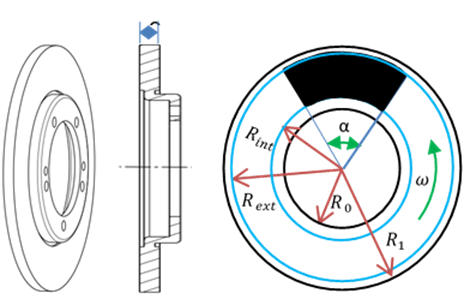Figure 1. Geometry of disc 5. Hypotheses Thanks to the axial symmetry, the geometric domain of the study was reduced to half. Thermo physical parameters: volume density, heat capacity, thermal conductivity, and thermal capacity are considered to be variable and temperature-dependent. The materials composing the disc and the pads are assumed to be homogeneous and isotropic. The contact between the disc and the pads is assumed to be perfect. During braking, the average angular speed of rotation is very high, the contact in the zone of friction between the disc and the pads is assumed to be permanent. And the circumferential gradient of temperature is zero. 6. Physical Modeling of the Heat Transfer Problem 6.1 Calculation domain The brake disc is modeled in three dimensions by a full disc with simplified geometry and real dimensions. represented by the Figure 2. ## 2.pngFigure 2. Geometric model of disc brake 6.2 Equation According to Maniana et al.  in 2009, the phenomenon of heat transfer by conduction-convection and boundary conditions in materials, where thermophysical characteristics, vary with temperature and distance, is modeled in the different elements of the brake by the Eqns. (20), (21), (22) and (23). \rho(T) c(T) \frac{\partial T(r, t)}{\partial t} \operatorname{div}(-k(T) \overrightarrow{g r a d}(T(r, t)))$$=\dot{q}(r, t)$      (20)

where, the flux φ(r, t) is considered as a surface heat source $\dot{q}(r, t)$ applied to the contact surface.

6.3 Boundary conditions, case of disc

On the total surface.

$-k_{d} \overrightarrow{\operatorname{grad}}\left(T_{d}\right)=h_{d}\left(T_{d}-T_{\text {ext }}\right)$     (21)

6.4 Boundary conditions, case of Pad

On the friction surfaces.

$-k_{g} \overrightarrow{\operatorname{grad}}\left(T_{g}\right)=\overrightarrow{0}$      (22)

On the rest of the free surface in contact with the air:

$-k_{g} \overrightarrow{\operatorname{grad}}\left(T_{g}\right)=h_{g}\left(T_{g}-T_{e x t}\right)$      (23)

6.5 Forced convection

The calculation of the convection coefficient (h) depends on the nature of the surface in contact with the air flow.

The most weighted convection is that of the lateral surface of the disc for which one can use the empirical relation of Morgan and Denis  to calculate the Nusselt number for the rotating brake disc Eq. (24).

A factor (A) which takes the reduction of the Nusselt number, due to the influence of the brake pads, into consideration.

$N u=A \times 0.0436 \times\left(\frac{R e}{R e_{\omega}}\right)^{0.74} \times R e_{\omega}^{0.8}$      (24)

The reduction factor (A) depends on the angle (α) of the Pad geometry . This correspondence is plotted in Figure 6.

$\alpha=\frac{\pi}{3} \rightarrow A=0.9$      (25)

Reynolds number: $R e=\frac{V(t) \cdot r}{v}$      (26)

Rotating Reynolds: $R e_{\omega}=\frac{\omega(t) \cdot r^{2}}{v}$      (27)

$h=\frac{k_{a i r}}{r} . N u$      (28)

In Table 1 we define all the thermophysical parameters of the air necessary for the calculation.

Table 1. Air characteristics

 Characteristic Value Temperature Kinematic viscosity 15.6 × 10−6 25℃ Volumetric density 1.184 25℃ Thermal conductivity 0.0262 20℃

6.6 Meshing

The disc and pad are meshed by linear cubic with eight nodes, see Figures 3. the results of the mesh are explored in Table 2.

## 3.pngFigure 3. Mesh

Table 2. Elements of mesh

 Number of Disc pad Element 43140 2560 Nod 50205 43140

6.7 Applied heat flux model

The heat flux distribution applied to the contact surface varies with radius and with time, it is modeled by Eq. (29) and plotted in Figure 4.

$\varphi_{d}=p \times \emptyset_{0} \times \emptyset(r) \times\left(1-\frac{t}{t_{f}}\right)$       (29)

## 4.png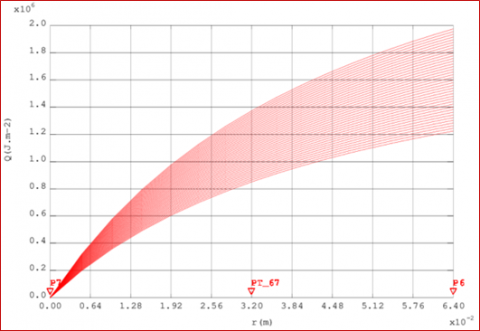Figure 4. (φd) Thermal source applied versus radius and time

6.8 Data of the problem

The numerical data of this thermal transfer problem in the disc brake are defined in Table 3 :

Tout have a good precision in the numerical calculation the physical parameters of the disc: volumic density (r), specific heat capacity (c), thermal conduction coefficient (k) and the friction coefficient (μ) in the contact disc/pad are considered variable with temperature. The curves are chon in Figure 5.

Table 3. Parameters of automotive brake application

 Setting Disc Pad Density (kg.m-3) Mass thermal capacity: c (J.kg-1. K-1) Conductivity: k (W.m-1.K-1) External Contact Diameter: dext (mm) Internal contact Diameter: dint (mm) Thickness: e (mm) Surface (m2) 7800-8600 450-850 35-55 228 100 11 3.29 10-2 2000 900 12 228 100 8 0.548 10-2 Angle that limits the fitting: α (rad) Coefficient of friction μ π/3 0.21-0.46 Braking time: tb (s) Total energy to be dissipated ft(J) Masse of vehicle M (kg) Vehicle wheel radius R (mm) Initial speed of vehicle V0 (m.s-1) Initial angular velocity ω0 (rad. s-1) Deceleration a (m.s-2) Convection coef.: h (W.m-2. K-1) Pressure P (MPa) 3.96 184.32 103 1590 324 27.8 85.8 7.02 20-300 1.2

## 5a.png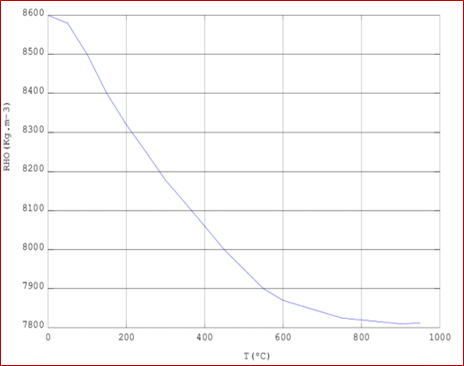(ρ) Volumic density

## 5b.png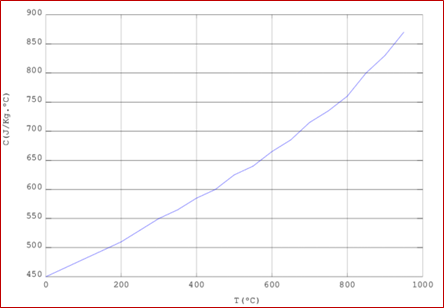(c) Specific heat capacity

## 5c.png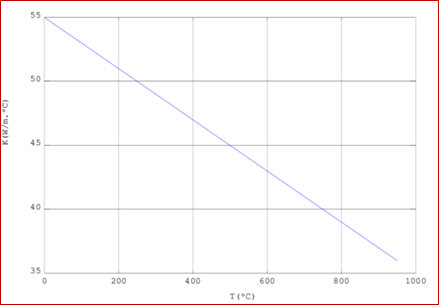(k) Thermal conduction coefficient

## 5d.png($\mu$) Friction coefficient

Figure 5. Thermophysical parameters

## 6.pngFigure 6. (A) Reduction factor

7. Results

In Figure 7, we represent the analytical solutions of Newcomb and Limpert. And in Figure 8, we compare the numerical solution (Average temperature at the friction surface) to the analytical Newcomb solution.

## 7.pngFigure 7. Analytical solutions

## 8.png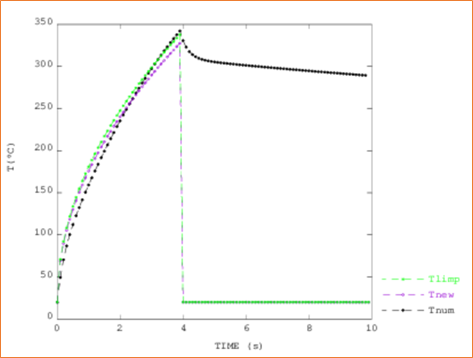Figure 8. Analytical and numerical

In the following graph (Figure 9), the radial distribution of temperature maximum versus radius on disc surface, calculated by the numerical simulation, is plotted.

## 9.png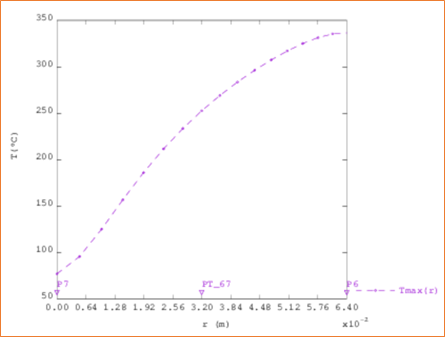Figure 9. Radial distribution of maximum temperature versus time

In Figure 7 we have represented the analytical solution (evolution of the maximum temperature versus time at a point on the disc/pad contact surface). In Figure 10 we have represented the evolution of the temperature versus time at three points distinct from the friction surface.

If one makes a comparative study between the two solutions in note that the temperature in the external radius coincides perfectly with the analytical solution. And that the latter can only inform us about the maximum temperature and on top of that it assumes that the temperature is uniform over the entire contact surface.

On the other hand, the numerical study shows that the profile of the temperature is variable according to the radius of the disc Figure 9.

## 10.png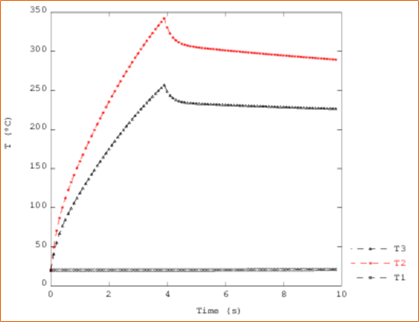Figure 10. Disc surface temperature at different radial distances with numeric method

On the graph of Figure 11 we have represented the initial distribution of the heat flow applied on the disc.

## 11.png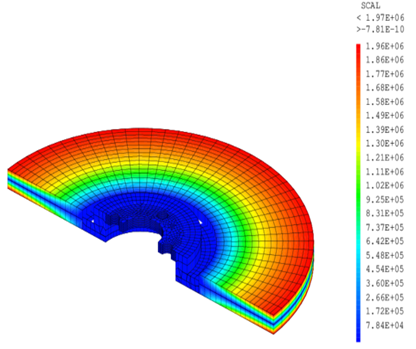Figure 11. Density heat flow initially applied to disc

In Figure 12 we represent the distribution of the maximum temperature, reached at the end of the braking period, in the disc by the isothermal surfaces.

## 12.png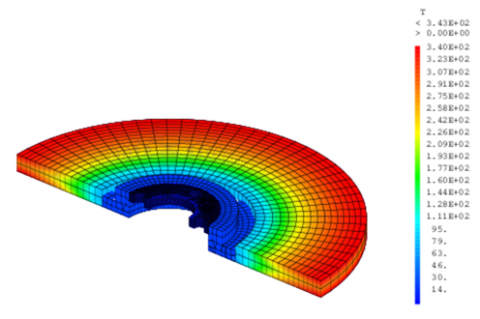Figure 12. Isovaleurs of temperature in disc

8. Conclusions

The comparison between the approximate analytical solutions of Newcomb and Limpert, drawn in the Figure 7, for the estimation of the maximum temperature at the contact surface between the disc and the pad gives a good agreement.

We notice a very small difference between the numerical and the analytical solution one in the estimation of the maximum temperature at the surface, this is said when taking into account the evolution of the thermo-physical parameters as a function of the temperature of on the one hand and the taking into account of convection on the other hand in the numerical model.

Nomenclature
 α Angle of pads (rad) ∅ Heat flow (W) φ Surface heat flow (W.m-2) k Conductivity (W.m-1.K-1) μ Coefficient of friction (without dimension) ρ Density (kg.m-3) ξ Thermal effusivity = $\sqrt{\mathrm{k} \rho \mathrm{c}}$ (J.m-2.K-1.s-0.5) $v$ kinematic viscosity of air (m2.s-1) a Thermal diffusivity = $\frac{\mathrm{k}}{\rho \mathrm{c}}$ (m2.s-1) c Mass calorific capacity (mass heat) (J.kg-1.K1) h Convection coefficient (W.m-2.K-1) t Time (s) tbr Braking time (s) tf final time (s) Nu Number of Nusselt (-) Re Number of Reynolds (-) P Pressure (Pa) p Partition Flow coefficient between disc and pad (-) q Volumetric Heat Flow (W.m-3) r Contact Radius (m) S Surface (m²) T Temperature (°C) V(t) Speed (m.s-1) V Volume (m3) ω(t) Angular velocity (rad.s-1) e Thickness (m) Subscripts d Disc g Garniture (pad) f. Final ini Initial int Internal ext External t Total b Braking
References

 Majcherczak, D., Dufrenoy, P., Naıt-Abdelaziz, M. (2005). Third body inﬂuence on thermal friction contact problems: Application to braking. Journal of Tribology, 127(1): 89-95. https://doi.org/10.1115/1.1757490

 Newcomb, T.P. (1959). Transient temperatures attained in disk brakes. British Journal of Applied Physics, 10(7): 339-340. https://doi.org/10.1088/0508-3443/10/7/311

 Limpert, R. (2011). Brake Design and Safety. SAE International.

 Olesiak, Z., Pyryev, Y., Yevtushenko, A. (1997). Determination of temperature and wear during braking. Wear, 210(1-2): 120-126. https://doi.org/10.1016/S0043-1648(97)00086-0

 Vernotte, P. (1956). Calcul numérique, calcul physique, application à la thermocinétique (Vol. 319). En vente au Service de documentation et d'information technique de l'aéronautique.

 Travaglia, C.A.P. (2014). Análise das Tensões Térmicas e Mecânicas no Material de Atrito de Veículos Comerciais Equipados com Tambores de Freio Através do Método de Elementos Finitos. Mastering Thesis, UFF–Universidade Federal Fluminense, Volta Redonda, RJ. https://doi.org/10.4236/eng.2014.613092

 Talati, F., Jalalifar, S. (2009). Analysis of heat conduction in a disk brake system. Heat and Mass Transfer, 45(8): 1047-1059. https://doi.org/10.1007/s00231-009-0476-y

 Hohmann, C., Schiffner, K., Oerter, K., Reese, H. (1999). Contact analysis for drum brakes and disk brakes using ADINA. Computers & Structures, 72(1-3): 185-198. https://doi.org/10.1016/S0045-949(99)00007-3.

 Maniana, M., Azim, A., Rhanim, H., Archambault, P. (2009). Problème inverse pour le traitement laser des métaux à transformations de phases. International Journal of Thermal Sciences, 48(4): 795-804. https://doi.org/10.1016/j.ijthermalsci.2008.05.018

 Morgan, S., Dennis, R.W. (1972). A theoretical prediction of disc brake temperatures and a comparison with experimental data (No. 720090). SAE Technical Paper. https://doi.org/10.4271/72